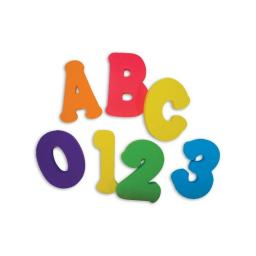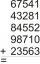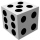# Divisibility by 12

Replace the letters A and B by digits so that the resulting number x is divisible by twelve /find all options/.

x = 2A3B

How many are the overall solutions?

n =  6

### Step-by-step explanation:

A=1, B=6, x=2136; x/12 = 178
A=2, B=2, x=2232; x/12 = 186
A=4, B=6, x=2436; x/12 = 203
A=5, B=2, x=2532; x/12 = 211
A=7, B=6, x=2736; x/12 = 228
A=8, B=2, x=2832; x/12 = 236Did you find an error or inaccuracy? Feel free to write us. Thank you!## Related math problems and questions:

• By sixFrom the digits 1,2,3,4 we create the long integer number 123412341234. .. .. , which will have 962 digits. Is this number divisible by 6?
• SymmetryEva loves symmetry in shapes and numbers. Yesterday she invented a completely new kind of symmetry - divisible symmetry. She wrote all five-digit numbers with different digits with the following property: the first digit is divisible by 1, the second by 2
• Divisible by nineHow many three-digit natural numbers in total are divisible without a remainder by the number 9?
• Find unknown numberWhat is the number between 50 and 55 that is divisible by 2,3,6,9?Fill letters instead of digits so the indicated sum (equal letters represent equal digits). What number is hidden under the letter J? A A H A H O A H O J -------------------------- 4 3 2 1
• Unknown integerFind the smallest integer: divided by 2, the remainder is 1. divided by 3, the remainder is 2. divided by 4, the remainder is 3. ... divided by eight, the remainder is 7, divided by 9 the remainder is 8.
• Three digits numberFrom the numbers 1, 2, 3, 4, 5 create three-digit numbers that digits not repeat and number is divisible by 2. How many numbers are there?
• NumberWhat number should be placed instead of the asterisk in number 702*8 to get a number divisible by 6?
• Three digits number 2Find the number of all three-digit positive integers that can be put together from digits 1,2,3,4 and which are subject to the same time has the following conditions: on one positions is one of the numbers 1,3,4, on the place of hundreds 4 or 2.
• The diceFind the probability that a number divisible by two or three will fall when the dice are rolled.
• Three-digit numbersHow many are all three-digit numbers that are made up of digits 0,2,5,7 and are divisible by 9 if the digits can be repeated?
• The largest numberFind the largest integer such that: 1. No figures is not repeat, 2. multiplication of every two digits is odd, 3. addition all digits is odd.
• What are 3What are the two digits which when inserted in the blank spaces will make 234 _ _ divisible by 8?
• 7 digit numberIf 3c54d10 is divisible by 330, what is the sum of c and d?
• Dd 2-digit numbersFind all odd 2-digit natural numbers compiled from digits 1; 3; 4; 6; 8 if the digits are not repeated.
• Six-digit primesFind all six-digit prime numbers that contain each one of digits 1,2,4,5,7, and 8 just once. How many are they?
• Prime divisorsFind 2/3 of the sum's ratio and the product of all prime divisors of the number 120.